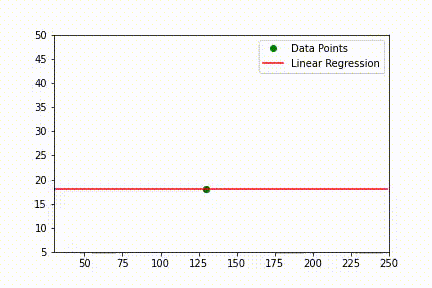# What does it mean by fitting a model in Machine Learning?

In Machine Learning, fitting a model to a dataset is synonymous to training a model. By fitting we mean learning the parameters of a model using the training dataset and these parameters helps defining the mathematical formulas behind the machine learning model.Learning of a Linear Regression model with introduction of additional data.

### Learning Linear Regression Parameters

Let's take a simple linear regression model of the form: y = ax + b
Here,
• y is the prediction to be made by the model
• x is the input data
• a and b are the parameter.
The model will make a correct decision only when all the parameters are at their best value. Since inputs (x) are the values we observed from real world we can't change the value of input. So only the value of a and b are to be changed to make correct prediction.

### Code Example

from sklearn import datasets
from sklearn.linear_model import LinearRegression